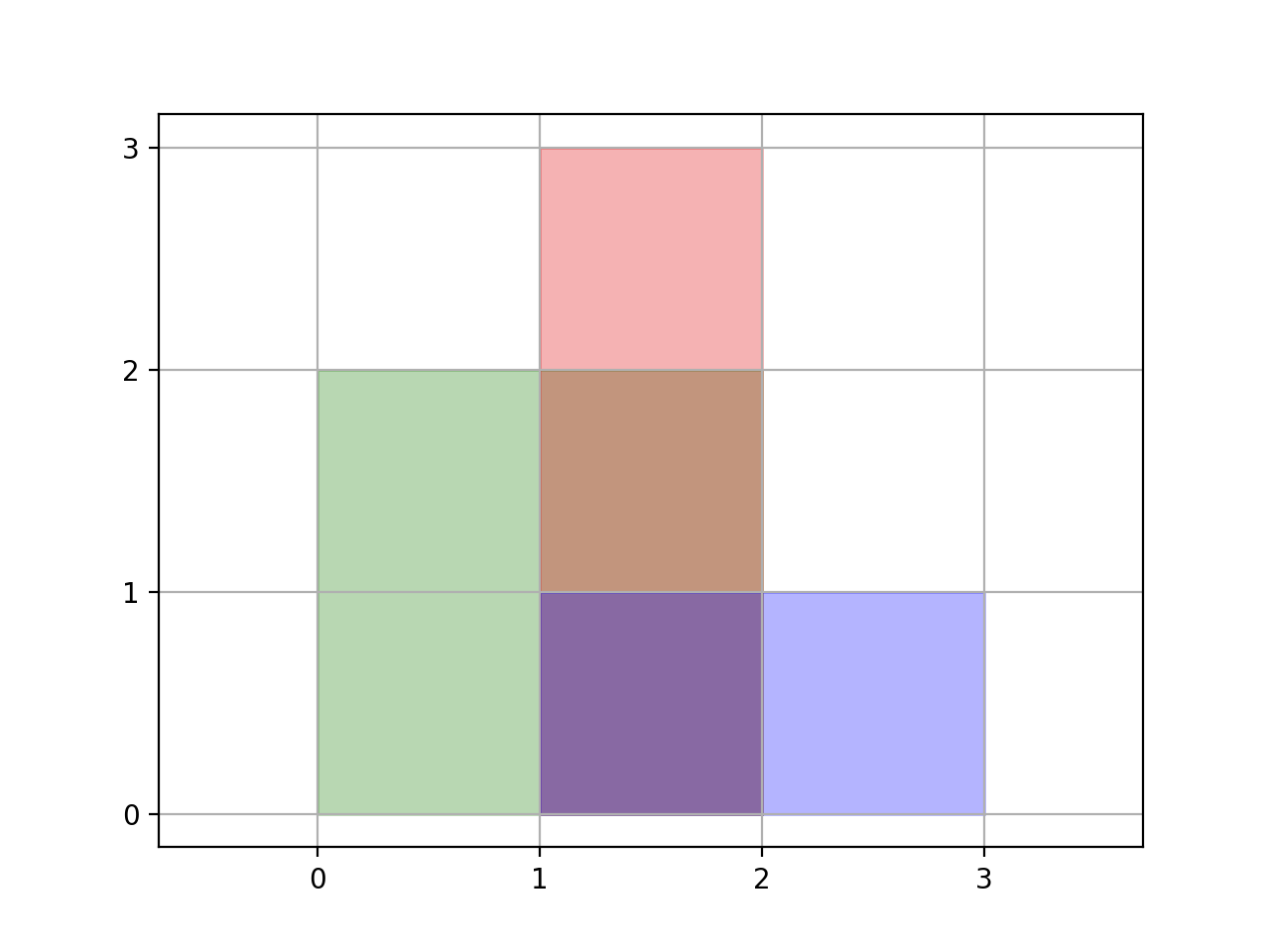Formatted question description: https://leetcode.ca/all/850.html

# 850. Rectangle Area II

Hard

## Description

We are given a list of (axis-aligned) rectangles. Each rectangle[i] = [x1, y1, x2, y2], where (x1, y1) are the coordinates of the bottom-left corner, and (x2, y2) are the coordinates of the top-right corner of the ith rectangle.

Find the total area covered by all rectangles in the plane. Since the answer may be too large, return it modulo 10^9 + 7.Example 1:

Input: [[0,0,2,2],[1,0,2,3],[1,0,3,1]]

Output: 6

Explanation: As illustrated in the picture.

Example 2:

Input: [[0,0,1000000000,1000000000]]

Output: 49

Explanation: The answer is 10^18 modulo (10^9 + 7), which is (10^9-(10^9+7))^2 = (-7)^2 = 49.

Note:

• 1 <= rectangles.length <= 200
• rectanges[i].length = 4
• 0 <= rectangles[i][j] <= 10^9
• The total area covered by all rectangles will never exceed 2^63 - 1 and thus will fit in a 64-bit signed integer.

## Solution

Loop over rectangles and use a tree set to store all x-axis values in ascending order without duplicates. Then loop over all x-axis values in reversing order. Each time obtain two adjacent x-axis values. Loop over rectangles. If a rectangle’s x-axis values are in the range of the two adjacent x-axis values, obtain the ranges in the direction of y-axis and add the area to the total area. Finally, return the total area.

class Solution {
public int rectangleArea(int[][] rectangles) {
final int MODULO = 1000000007;
TreeSet<Integer> xSet = new TreeSet<Integer>();
long area = 0;
for (int[] rectangle : rectangles) {
}
List<Integer> xList = new ArrayList<Integer>(xSet);
for (int i = xList.size() - 2; i >= 0; i--) {
List<int[]> yList = new ArrayList<int[]>();
for (int[] rectangle : rectangles) {
if (xList.get(i) >= rectangle && xList.get(i + 1) <= rectangle) {
int[] interval = {rectangle, rectangle};
yList = insert(yList, interval);
}
}
long distance = 0;
for (int[] interval : yList)
distance += interval - interval;
area = (area + (xList.get(i + 1) - xList.get(i)) * distance) % MODULO;
}
return (int) area;
}

public List<int[]> insert(List<int[]> intervals, int[] newInterval) {
List<int[]> intervalsList = new ArrayList<int[]>();
int start = newInterval, end = newInterval;
int size = intervals.size();
boolean inserted = false;
for (int i = 0; i < size; i++) {
int[] interval = intervals.get(i);
if (inserted)
else {
if (interval < start)
else if (interval > end) {
int[] insertInterval = {start, end};
inserted = true;
} else {
start = Math.min(start, interval);
end = Math.max(end, interval);
}
}
}
if (!inserted) {
int[] insertInterval = {start, end};